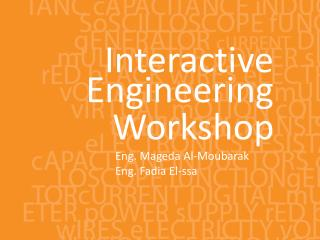DownloadDownload PresentationInteractive

# Interactive

Télécharger la présentation## Interactive

- - - - - - - - - - - - - - - - - - - - - - - - - - - E N D - - - - - - - - - - - - - - - - - - - - - - - - - - -
##### Presentation Transcript

1. Engineering Interactive Workshop Eng. Mageda Al-Moubarak Eng. Fadia El-ssa

2. Contents • Electric Elements • Instruments

3. Active Elements • Active elements are capable of providing an average power greater than zero to external devices, over an infinite period of time. • Examples Current source: produces a current in a conductor Voltage source: produces a potential difference between two points.

4. Passive Elements • Passive element is an element of the electrical circuit that Does Not create Power. Resistors, Capacitors, Inductors and diodes are examples of passive elements.

5. Voltage and Current • Voltage: A measure of the work to move a charge. Voltage= Energy/ Charge. • Current: Charge in motion Current= Charge/ Time. • Power = V*I= Energy * Charge = Energy Charge Time Time

6. Resistor • When a voltage V is applied across the terminals of a resistor, a current I will flow through the resistor. • The current is directly proportional to the voltage using ohm’s law I=1*V • R • Where the slope of the line = 1/R

7. Series Parallel

8. Shorted Resistor

9. Potentiometer

10. Capacitor • Capacitance C, measured in Farads - is an electronic component consisting of a pair of conductors separated by adielectric(insulator). • When there is a voltage across the conductors, a static electric field develops, that stores finite amount of energy. • Impedance Zc= 1/jωc, Where ω = 2πf.

11. Series Parallel

12. In the capacitor the Current leads the Voltage.

13. Inductor • Inductance L, measured in Henries –Typically an inductor is a conducting wire shaped as a coil. • electric current passing through the inductor creates a magnetic field and thus stores energy. • Impedance ZL= jωL , where ω = 2πf.

14. Series Parallel

15. In the inductor the Voltage leads the Current.

16. CIVIL

17. Diode • A diode is a two-terminal electronic component that conducts electric current in only one direction. • The most common function of a diode is to allow an electric current to pass in one direction while blocking current in the opposite direction.

18. Light Emitting Diode (LED)

20. Power Supply

21. Digital Multi-Meter (DMM)

22. Function Generator

23. Cathode Ray Oscilloscope

24. THE END

25. D. The Impedance of the Capacitor depends on the input’s frequency. TRUE

26. 1. Give 3 ways in which you can have an Equivalent Resistance of 60 KΩ. Given the following resistances, Two 1.2 * 105Ω 20 K Ω 12 K Ω 50 K Ω potentiometer. 47 K Ω 1 K Ω • Two 1.2 * 105Ω in parallel • 20 K Ω and 40 from the 50 K Ω potentiometer in series. • 12 K Ω, 47 K Ω, and 1 K Ω in Series.

27. 2.Design a circuit consisting of 2 resistors and a power supply of 9 V, whose Current is 3mA.

28. Using the Following Color Code. What is the value of this resistor? 82000Ω with ±5%

29. 5. How can you discharge a capacitor? Connecting it with a resistor

30. LED stands for …………………… Light Emitting Diode

31. B. Digital Multi-meter is used to measure ……………………………, …………………………, and ……………………… Resistance Voltage Current

32. C. DC offset Knob is found in an instrument which is ………………………… Function Generator

33. E. To generate a Square Wave. We Should use a(n) ………………………………. Function Generator

34. Given a 10K Potentiometer, and a fixed power supply of 5 V. Draw a circuit to show how you can get 3 V output. 5* R2 = 3V 10K R2= 6KΩ R1=10K-6K=4K Ω